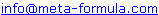# Metastock Formulas - Z Click here to go back to Metastock Formula Index

 IMPORTANT: These formulas aren't my complete collection. For my complete collection of instantly usable, profitable and powerful MetaStock formulas Click Here

Would You Like To Use MetaStock To Its Full Potential? Discover the Amazingly Simple Secret to Master Metastock Step-By-Step - click here

A | A 1 | B | B 1 | C | C 1 | D | D 1 | E | F | G | H | I | J | K | L | M | M 1 | N | O | P | R | S | S 1 | T | U | V | W | Z |

 Zero Lag EMA Zero Lag MACD Zero Lag MACD Trigger Signal Zig Zag Validity Zigzag Validity Indicator ZigZag - Hi/Lo

## Zero Lag EMA

Here's my Metastock 6.2 coded version of the Zero Lag Moving Average, as described in the April, 2000, issue of Technical Analysis of Stocks and Commodities. I've also used it to construct a Zero Lag MACD and a Zero Lag MACD trigger signal.

Period:= Input("What Period",1,250,10);
EMA1:= Mov(CLOSE,Period,E);
EMA2:= Mov(EMA1,Period,E);
Difference:= EMA1 - EMA2;
ZeroLagEMA:= EMA1 + Difference;
ZeroLagEMA

(Go Top)

## Zero Lag MACD

EMA1:= Mov(CLOSE,13,E);
EMA2:= Mov(EMA1,13,E);
Difference:= EMA1 - EMA2;
ZeroLagEMA13:= EMA1 + Difference;
EMA1:= Mov(CLOSE,21,E);
EMA2:= Mov(EMA1,21,E);
Difference:= EMA1 - EMA2;
ZeroLagEMA21:= EMA1 + Difference;
ZeroLagMACD:=ZeroLagEMA13 - ZeroLagEMA21;
ZeroLagMACD

(Go Top)

## Zero Lag MACD Trigger Signal

(To be used with the ZeroLag MACD above)

EMA1:= Mov(CLOSE,13,E);
EMA2:= Mov(EMA1,13,E);
Difference:= EMA1 - EMA2;
ZeroLagEMA13:= EMA1 + Difference;
EMA1:= Mov(CLOSE,21,E);
EMA2:= Mov(EMA1,21,E);
Difference:= EMA1 - EMA2;
ZeroLagEMA21:= EMA1 + Difference;
ZeroLagMACD:=ZeroLagEMA13 - ZeroLagEMA21;
EMA1:= Mov(ZeroLagMACD,8,E);
EMA2:= Mov(EMA1,8,E);
Difference:= EMA1 - EMA2;
ZeroLagTRIG:= EMA1 + Difference;
ZeroLagTRIG

(Go Top)

## Zig Zag Validity

perc:=Input("Percent",2,100,10);
Z:=Zig(C,perc,%);
last:=ValueWhen(1,
( Z > Ref(Z,-1) AND Ref(Z,-1) < Ref(Z,-2) )
OR
( Z < Ref(Z,-1) AND Ref(Z,-1) > Ref(Z,-2) ),
Ref(Z,-1));
pc:=(C-last) * 100 / last;
pc:= Abs(pc);
SD:=(z>Ref(z,-1) AND Ref(z,-1)>Ref(z,-2)) OR (z<Ref(z,-1) AND
Ref(z,-1)<Ref(z,-2));
res:=If(pc>=perc ,1,0);

(Go Top)

## Zigzag Validity Indicator

Spyros Raftopoulos

1=valid last leg of zig,

-1=invalid last leg of zig.

DO NOT use this indicator in systems}

perc:=Input("Percent",0.2,100,10);

Z:=Zig(C,perc,%);

last:=ValueWhen(1,

( Z > Ref(Z,-1) AND Ref(Z,-1) < Ref(Z,-2) )

OR

( Z < Ref(Z,-1) AND Ref(Z,-1) > Ref(Z,-2) ),

Ref(Z,-1));

pc:=(C-last) * 100 / last;

pc:= Abs(pc);

SD:=(z>Ref(z,-1) AND Ref(z,-1)>Ref(z,-2)) OR (z<Ref(z,-1) AND

Ref(z,-1)<Ref(z,-2));

res:=If(pc>=perc ,1,0);

res

(Go Top)

## ZigZag - Hi/Lo

{ High/Low ZigZag v1.0 }{ Plot on price chart }{ ©Copyright 2004 Jose Silva }{ [email protected] }

pr:=Input("ZigZag reversal amount", 0.001,100000,5);
choose:=Input("Hi/Lo ZigZag,  Close ZZ,  Peaks/Troughs",1,3,1);

pk:=PeakBars(1,H,pr)=0;
tr:=TroughBars(1,L,pr)=0;
pktr:=pk-tr;

zz:=Zig(C,pr,%);
zzHi:=Zig(H,pr,%);
zzLo:=Zig(L,pr,%);
avg:=(zzHi+zzLo)/2;

x:=
If(pk,zzHi,If(tr,zzLo,If(avg>Ref(avg,-1),H,L)));
zzHiLo:=Zig(x,pr,%);

If(choose=1,zzHiLo,If(choose=2,zz,pktr))

(Go Top)

If you have Metastock formulas you would like to share,
Please email toWe look forward to hearing from you!